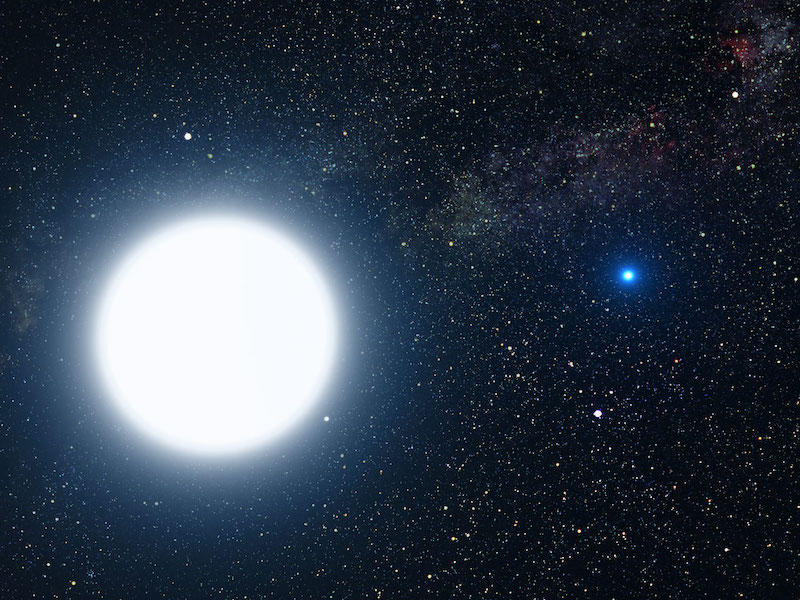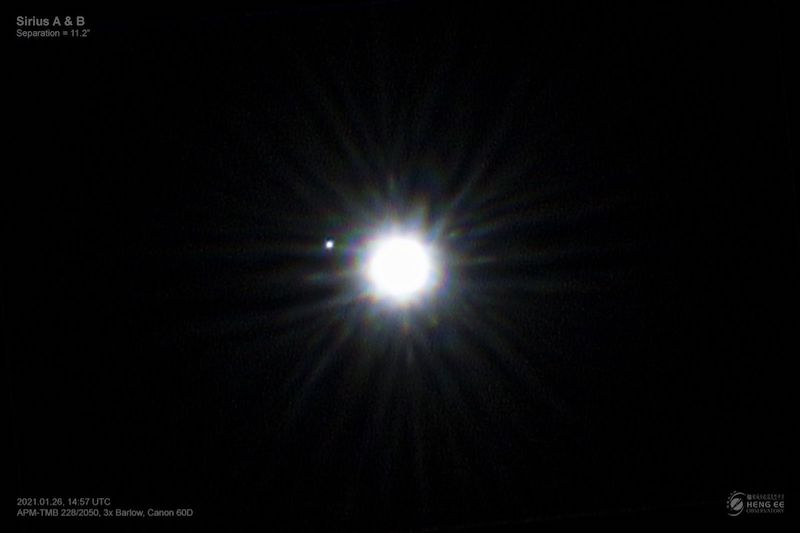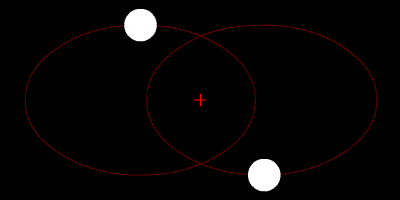Human WorldSpace

# How do you measure the mass of a star?Artist’s concept of the binary star system of Sirius A and its small blue companion, Sirius B, a hot white dwarf. The 2 stars revolve around each other every 50 years. Binary stars are useful to determine the mass of a star. Image via ESA/ G. Bacon.

### To measure the mass of a star, use 2 stars

There are lots of binary stars – two stars revolving around a common center of mass – populating the starry sky. In fact, a large majority of all stars we see (around 85%) are thought to be part of multiple star systems of two or more stars! This is lucky for astronomers, because two stars together provide an easy way to measure star masses.

To find the masses of stars in double systems, you need to know only two things. First, the semi-major axis or mean distance between the two stars (often expressed in astronomical units, which is the average distance between the Earth and sun).

And second, you need to know the time it takes for the two stars to revolve around one another (aka the orbital period, often expressed in Earth years).

With those two observations alone, astronomers can calculate the stars’ masses. They typically do that in units of solar masses (that is, a measure of how many of our suns the star “weighs.” One solar mass is 1.989 x 1030 kilograms or about 333,000 times the mass of our planet Earth.)

Available now! 2023 EarthSky lunar calendar. A unique and beautiful poster-sized calendar showing phases of the moon every night of the year! And it makes a great gift.

### Sirius is a great example

We’ll use Sirius, the brightest star of the nighttime sky, as an example. It looks like a single star to the unaided eye, but it, too, is a binary star. By the way, you can see it yourself, if you have a small telescope.

The two stars orbit each other with a period of about 50.1 Earth-years, at an average distance of about 19.8 astronomical units (AU). The brighter of the two is called Sirius A, while its fainter companion is known as Sirius B (The Pup).View at EarthSky Community Photos. | Michael Teoh at Heng Ee Observatory in Penang, Malaysia, captured this photo of Sirius A and Sirius B (a white dwarf) on January 26, 2021. He used 30 1-second exposures and stacked them together to make faint Sirius B appear. Thank you, Michael!

### Finding the mass of Sirius A and B

So how would astronomers find the masses of Sirius A and B? They would simply plug in the mean distance between the two stars (19.8 AU) and their orbital period (50.1 Earth-years) into the easy-to-use formula below, first derived by Johannes Kepler in 1618, and known as Kepler’s Third law:

Total mass = distance3/period2
Total mass = 19.83/50.12
So total mass = 7762.39/2510.01 = 3.09 times the sun’s mass

Here, the distance is the mean distance between the stars (or, more precisely, the semi-major axis) in astronomical units, so 19.8, and the orbital period is 50.1 years.

The resulting total mass is about three solar masses. Note that this is not the mass of one star but of both stars added together. So, we know that the whole binary system equals three solar masses.An example of a binary star system, whose component stars orbit around a common center of mass (the red cross). In this depiction, the two stars have similar masses. In the case of the Sirius binary star system, Sirius A has about twice the mass of Sirius B. Image via Wikimedia Commons.

### Then finding the mass of each star

To find out the mass of each individual star, astronomers need to know the mean distance of each star from the barycenter: their common center of mass. To learn this, once again they rely on their observations.

It turns out that Sirius B, the less massive star, is about twice as far from the barycenter than is Sirius A. That means Sirius B has about half the mass of Sirius A.

Thus, you know the whole system is about three solar masses by using Kepler’s Third Law. So now you can deduce that the mass of Sirius A is about two solar masses. And then Sirius B pretty much equals our sun in mass.

### What about the mass of a star not in a binary system?

But what about stars that are alone in their star systems, like the sun? The binary star systems are once again the key: Once we have calculated the masses for a whole lot of stars in binary systems, and also know how luminous they are, we notice that there is a relationship between their luminosity and their mass. In other words, for single stars we only need to measure its luminosity and then use the mass-luminosity relation to figure out their mass. Thank you, binaries!

Read more: What is stellar luminosity?

Read more: What is stellar magnitude?

Bottom line: For astronomers, binary star systems are a quite useful tool to figure out the mass of stars.

Posted
January 15, 2023
in
Human World